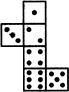# Non Verbal Reasoning - Cubes and Dice - Discussion

### Discussion :: Cubes and Dice - Section 1 (Q.No.20)

The sheet of paper shown in the figure (X) given on the left hand side, in each problem, is folded to form a box. Choose from amongst the alternatives (1), (2), (3) and (4), the boxes that are similar to the box that will be formed.

20.

When the following figure is folded to form a cube, how many dots lie opposite the face bearing five dots?[A]. 1 [B]. 2 [C]. 3 [D]. 4

Explanation:

The given figure is similar to Form III. Therefore, when this figure is folded to form a cube then the face bearing three dots will lie opposite the face bearing five dots.

 Anonym said: (Oct 3, 2020) To find the opposite faces just look for a box which is not adjacent (attached directly) to that box and at a distance of one box away.